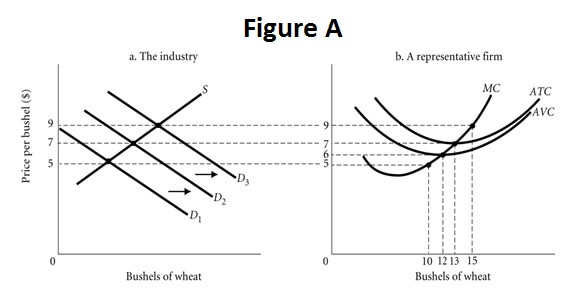# Perfect Competition Costs and Profit Maximization Assignment Help

QuantityTFCTVCTC = TFC + TVCTR (when P=\$14)TR(when P=\$18)TR(when P=\$44)TR(when P=\$53)TR(when P=\$70)
04504500000
14520651418445370
2453580283688106140
34545904254132159210
445751205672176212280
5451201657090220265350
64518022584108264318420

## Perfect Competition Costs and Profit Maximization Assignment Help By Online Tutoring and Guided Sessions at AssignmentHelp.Net

Examine the table above, which gives information about the costs of a perfectly competitive firm. You are hired to determine the profit-maximizing quantity for the firm at different prices. For each price listed, you must find the output level, total revenue, total cost, and profit.

When the market price is P = \$14, the profit maximizing level of output is 3.

The total revenue at this level of output is 42. The total cost at this level of output is 90. The profit at this level of output is -48.

When the market price is P = \$18, the profit maximizing level of output is 2.

The total revenue at this level of output is 36. The total cost at this level of output is 80. The profit at this level of output is -44.

When the market price is P = \$44, the profit maximizing level of output is 4.

The total revenue at this level of output is 176. The total cost at this level of output is 120. The profit at this level of output is 56.

When the market price is P = \$53, the profit maximizing level of output is 5.

The total revenue at this level of output is 265. The total cost at this level of output is 165. The profit at this level of output is 100.

When the market price is P = \$70, the profit maximizing level of output is 6.

The total revenue at this level of output is 420. The total cost at this level of output is 225. The profit at this level of output is 195

If the average variable cost curve is above the marginal cost curve, then marginal costs can be either increasing or decreasing.

In the short run where total variable cost is increasing at a decreasing rate, marginal cost is positive and decreasing.

Total variable cost always increase with output.

Because marginal cost is always positive in the short run, total variable cost always increased when output increases.

If marginal cost is between average variable cost and average total cost, then average variable cost is increasing and average total cost is decreasing.

If we know average total cost and the amount of output, then we can always calculate total cost by multiplying average total cost by the amount of output.

The formula for AVC is TVC/q.

In the short run, firms act to minimize losses or maximize profits.

Firms that are breaking even are earning zero economic profits.

```{`
A profit-maximizing strategy becomes a loss minimization strategy when a firm in a perfectly competitive industry is producing where AVC < P < ATC.
A firm will choose to operate rather than shut down as long as price is greater than or equal to AVC.
Economic Profit is (P‐ATC)*q.
You are hired as an economic consultant to The Pampered Pet Shop. The Pampered Pet Shop operates in a perfectly competitive industry. This firm is currently producing at a point where market price equals its marginal cost. The shop’s total revenue exceeds its total variable cost, but is less than its total cost. You should advise the firm to produce in the short run to minimize its loss, but exit the industry in the long run.
A firm will shut down in the short run if variable costs exceed revenues.
A firm that is earning positive profits in the short run has an incentive to expand its scale of operation in the long run.
If revenues exceed variable cost, economic profit is positive.
A firm can minimize its losses by shutting down when revenues re less than variable costs.
The Taste Freeze Ice Cream Company is a perfectly competitive firm producing where MR = MC. The current market price of an ice cream sandwich is \$5.00. Taste Freeze sells 200 ice cream sandwiches. Its AVC is \$8.00 and its AFC is \$3.00. In this case Taste Freeze should shut down and produce zero sandwiches because price is less than AVC.`}```

Refer to Figure A. the firm continue to operate but at a loss when P is in range \$6-\$7. The firmapos;s shut down point is at a price of \$6.

Suppose demand for wheat is initially D2. If consumer incomes increase, then demand for wheat will shift to D3. Suppose demand for wheat is initially D2. If the price of rice (a substitute for wheat) falls, then demand for wheat will shift to D1. If demand for wheat is D2, then a profit maximizing firm will produce13 units and earn a profit of \$0. If demand for wheat is D3, then a profit maximizing firm will produce 15 units and earn positive profits. If demand for wheat is D1, then a profit maximizing firm will produce 0 units and earn negative profits. This firm will then exit the industry in the long run.

Suppose a monopolist faces the following demand curve: P = 596 – 4Q. The Marginal cost of production is constant and equal to \$60, and there are no fixed costs. What is the monopolist’s profit-maximizing level of output? What price will the profit-maximizing monopolist charge? How much profit will the monopolist make if she maximizes her profit? What would be the value of consumer surplus in this monopoly market? How much consumer surplus would there be if this market was perfectly competitive? What is the value of the deadweight loss when the market is a monopoly?